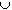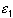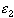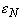#Interactive Real Analysis

Next | Previous | Glossary | Map

## 5.1. Open and Closed Sets

### Proposition 5.1.3: Unions of Open Sets, Intersections of Closed Sets

• Every union of open sets is again open.
• Every intersection of closed sets is again closed.
• Every finite intersection of open sets is again open
• Every finite union of closed sets is again closed.

### Proof:

Let { U n } be a collection of open sets, and let U =U n. Take any x in U. Being in the union of all U's, it must be contained in one specific U n. Since that set is open, there exists a neighborhood of x contained in that specific U n. But then that neighborhood must also be contained in the union U. Hence, any x in U has a neighborhood that is also in U, which means by definition that U is open.

To prove the second statement, simply use the definition of closed sets and de Morgan's laws.

Now let U n, n=1, 2, 3, ..., N be finitely many open sets. Take x in the intersection of all of them. Then:

• x is in the first set: there exists anwith ( x -, x +) contained in the first set
• x is in the second set: there iswith ( x -, x +) contained in the second set.
• ....
• x is in the N-th set: there iswith ( x -, x +) contained in the last set.
But then
• let= min{,, ...,}. Then ( x -, x +) is contained in each set U n
Hence it is contained in their finite intersection, which is therefore open, since x was arbitrary.

The last statement follows again from de Morgan's laws.Next | Previous | Glossary | Map